# GMAT Math : DSQ: Calculating the length of the diagonal of a rectangle

## Example Questions

### Example Question #1 : Dsq: Calculating The Length Of The Diagonal Of A Rectangle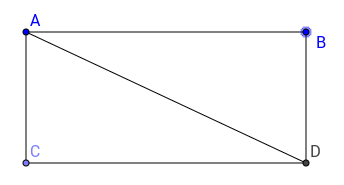What is the length of the diagonal of rectangle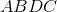(1)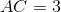(2)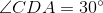and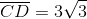Statement 2 alone is sufficient

Both statements together are sufficient

Statement 1 alone is sufficient

Each statement alone is sufficient

Statements 1 and 2 together are not sufficient

Statement 2 alone is sufficient

Explanation:

In order to find the diagonal, we must know the sides of the rectangle or know whether the triangles ADC or ABD have special angles.

Statement 1 alone doesn't let us calculate the hypothenuse of the triangles, because we only know one side.

Statement 2 alone is sufficient because it allows us to find all angles of the triangles inside of the rectangle. We can see that they are special triangles with angles 30-60-90. Any triangle with these angles will have its sides in ratio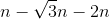, where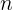is a constant. Here,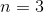, knowing this, we can calculate the length of the hypothenuse, also the diagonal, which will be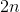.

Hence, statement 2 is sufficient.

### Example Question #2 : Dsq: Calculating The Length Of The Diagonal Of A Rectangle

Rectangle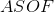has a perimeter of, what is its area?

I) The diagonal ofis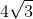inches.

II) The length of one side isinches.

Either statement alone is sufficient to answer the question.

Statement II is sufficient to answer the question, but Statement I is not sufficient to answer the question.

Both statements together are needed to answer the question.

Statement I is sufficient to answer the question, but Statement II is not sufficient to answer the question.

Statement II is sufficient to answer the question, but Statement I is not sufficient to answer the question.

Explanation:

I) Gives us the length of ASOF's diagonal. This by itself does not give us any way of finding the other sides.

II) Gives us one side length. From there we can use the perimeter to find the other side length and then the area.

Therefore, Statement II is sufficient to answer the question.

### Example Question #3 : Dsq: Calculating The Length Of The Diagonal Of A RectangleRectangle, has diagonal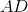. What is the length of?

(1) Angle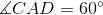.

(2)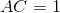.

Statement 2 alone is sufficient.

Statements 1 and 2 together are not sufficient.

Both statements together are sufficient.

Statement 1 alone is sufficient.

Each statement alone is sufficient.

Both statements together are sufficient.

Explanation:

The length of a diagonal of a rectangle can be calculated like a hypotenuse using the Pythagorean Theorem provided we have information about the lengths of the rectangle.

Statement 1 tells us that both triangles ADC and ABD have their angles in ratio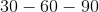, which means that their sides will have length in ratio, whereis a constant. We can't tell however what length the diagonal will be.

Statment 2 tells us that side AC is 1. From there we can't conclude anything. Indeed, rectangle ABCD might as well be a square or a very thin rectangle, we don't know.

Both statements together however, allow us to tell that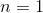and therefore that the diagonal will be 2.

Hence, both statements together are sufficient.

### Example Question #4 : Dsq: Calculating The Length Of The Diagonal Of A Rectangleis a rectangle. What is the ratio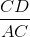?

(1)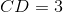.

(2)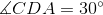.

Statement 1 and 2 together are not sufficient.

Each statement alone is sufficient.

Statement 1 alone is sufficient.

Both statements together are sufficient.

Statement 2 alone is sufficient.

Statement 2 alone is sufficient.

Explanation:

To solve this, we need information about the lengths of the sides or to whether triangles ADB and ACD are special triangles.

Statement 1 tells us that CDA has length 3. This is not enough and we still don't know whether the rectangle is of a special type of rectangle.

Statement 2 tells us that triangles ADB and ACD are special triangles, indeed, they have their angles in ratio. That means that their sides will be in ratio. Now we don't need to know what is constant, since it will cancel out in the ratio.

Therefore, statement 2 alone is sufficient.

### Example Question #5 : Dsq: Calculating The Length Of The Diagonal Of A Rectangle

Find the diagonal of rectangle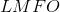.

I) The area ofis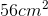.

II) The perimeter ofis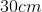.

Statement I is sufficient to answer the question, but statement II is not sufficient to answer the question.

Statement II is sufficient to answer the question, but statement I is not sufficient to answer the question.

Both statements are needed to answer the question.

Either statement is sufficient to answer the question.

Both statements are needed to answer the question.

Explanation:

In order to find the diagonal of a rectangle we need the length of both sides.

We can't find the lengths with just the area or just the perimeter, but by using them both together we can make a small system of equations with two unknowns and two equations.

Then we can solve for each side and use the Pythagorean Theorem to find our diagonal.

Tired of practice problems?

Try live online GMAT prep today.